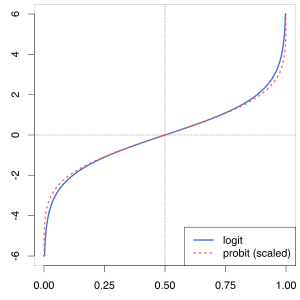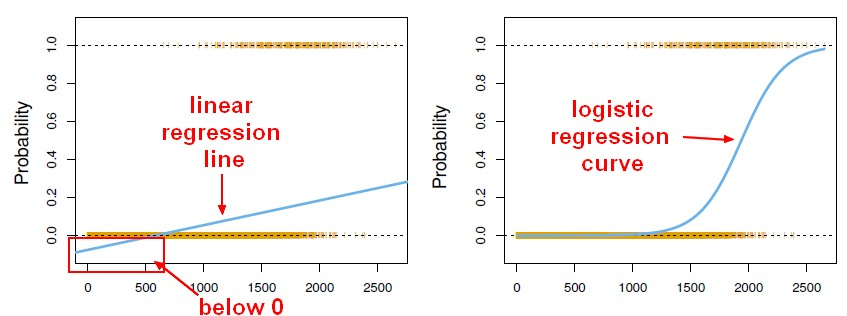# Statistics - Binary logistic regression

## Formula

$$\href{odds#logit}{Logit}(\hat{Y}) = ln(\frac{\hat{Y}}{1-\hat{Y}}) = B_0 + \sum_{i=1}^{k}{(B_iX_i)}$$

where:

• $\hat{Y}$ : predicted value on the outcome variable Y
• $Y$ : the outcome variable
• $B_0$ : predicted value on Y when all X = 0
• $X_i$ : predictor variables
• $B_i$ : unstandardized regression coefficients
• $Y-\hat{Y}$ : residual (prediction error)
• k = the number of predictor variables

## Logit Transformation

As:

• we're trying to predict an outcome variable that can only take on one of two values, 0 or 1.
• then we have to come up with a predicted score that falls between 0 and 1.

The general lineal model will not guarantee that the linear combination of the predictors will come up with a score that falls between 0 and 1. This is why the logit is used in the left hand site of the formula.

$$\href{odds#logit}{Logit}(\hat{Y}) = ln(\frac{\hat{Y}}{1-\hat{Y}})$$

The general lineal model assumes:

• a linear relationship between x and y,
• and a normal distribution in the outcome variable.

As the general lineal model will not work because we have a binary outcome variable, a logit transformation must be applied.

The logit transformation is a feature of an even more “general” mathematical framework in regression that is called the “Generalized Linear Model”. It sounds almost exactly the same as the general linear model but it's very different.

## Interpretation

For example with a coefficient regression B_1 of 0.39.

For every 1 unit increased in X, I'm going to predict:

• a 0,39 increase in the logit. (not directly in Y, therefore the scale is hard to interpret
• a 1,48 increase in the odds ratio.

Example for 1 unit increase:

• if the odds of X - 1, is 1.27, then the odds of X becomes: $1,27 * 1,48 = 1.88$
• in terms of probability, P(X)

$$\begin{array}{rrlrrl} P(X) & = & \frac{Odds}{1+Odds} \\ P(X) & = & \frac{1.88}{2.88} & = & .65 \\ P(X-1) & = & \frac{1.27}{2.27} & = & .56 \end{array}$$

## Hypothesis tests

We can look at:

• the individual predictors in the regression equation. Are they statistically significant?
• the overall model. Is the model statistically significant?
• model comparisons. Is model A significantly beter than model B?

### Individual Predictors

To test each predictor variable, we're going to look at:

• the regression coefficient
• the odds ratio
• the Wald test

#### Regression coefficient

Are they significant ?

#### Odds ratio

Odds ratio are more meaningful.

For one unit increase in X, the predicted changes in Odds.

It's also possible to report confidence interval for odds.

#### Wald test

Wald test test the model with the predictor versus a model without the predictor. The Wald test is very common in logistic regression, and in more advanced statistics. We can see how well does the model fit with the predictor in, and then with the predictor taken out.

The Wald test is a function of the regression coefficient. A wall test is calculated for each predictor variable and compares the fit of the model without the predictor.

### Overall Model

How to assess the overall fit of the model ?

#### Chi-square

Comparison of:

• a model with all the predictors
• to a chi-square that assumes no relationship between all the predictors and the outcome, otherwise known as the null model.

compare the fit of the model to the fit of the Null model.

#### Wald test

multiple models (Wald test)

#### Efficiency /Classification Success

Percentages of cases classified correctly.

See how well it classifies cases.

### Output Component

Main output component are:

• Regression Coefficient
• Odds Ratios
• Wald Tests
• Model Chi-Square
• Classification Success

Discover MoreData Mining - Probit Regression (probability on binary problem)

Probit_modelprobit model (probability + unit) is a type of regression where the dependent variable can only take two values. As the Probit function is really similar to the logit function, the probit...Machine Learning - (Univariate|Simple) Logistic regression

A Simple Logistic regression is a Logistic regression with only one parameters. For the generalization (ie with more than one parameter), see Logistic regression comes from the fact that linear regression...R - Generalized linear model (glm)

glm glm with the argument family equals binomial in order to apply the logit transformation. contingency tables or class tabs.